# Cycles

## Cycle double cover conjecture ★★★★

Author(s): Seymour; Szekeres

Conjecture   For every graph with no bridge, there is a list of cycles so that every edge is contained in exactly two.

Keywords: cover; cycle

## The circular embedding conjecture ★★★

Author(s): Haggard

Conjecture   Every 2-connected graph may be embedded in a surface so that the boundary of each face is a cycle.

Keywords: cover; cycle

## (m,n)-cycle covers ★★★

Author(s): Celmins; Preissmann

Conjecture   Every bridgeless graph has a (5,2)-cycle-cover.

Keywords: cover; cycle

## Faithful cycle covers ★★★

Author(s): Seymour

Conjecture   Ifis a graph,is admissable, and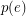is even for every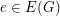, thenhas a faithful cover.

Keywords: cover; cycle

## Decomposing eulerian graphs ★★★

Author(s):

Conjecture   Ifis a 6-edge-connected Eulerian graph andis a 2-transition system for, thenhas a compaible decomposition.

Keywords: cover; cycle; Eulerian

## Barnette's Conjecture ★★★

Author(s): Barnette

Conjecture   Every 3-connected cubic planar bipartite graph is Hamiltonian.

Keywords: bipartite; cubic; hamiltonian

## r-regular graphs are not uniquely hamiltonian. ★★★

Author(s): Sheehan

Conjecture   Ifis a finite-regular graph, where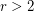, thenis not uniquely hamiltonian.

Keywords: hamiltonian; regular; uniquely hamiltonian

## Hamiltonian cycles in line graphs ★★★

Author(s): Thomassen

Conjecture   Every 4-connected line graph is hamiltonian.

Keywords: hamiltonian; line graphs

## Geodesic cycles and Tutte's Theorem ★★

Author(s): Georgakopoulos; Sprüssel

Problem   Ifis a-connected finite graph, is there an assignment of lengths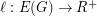to the edges of, such that every-geodesic cycle is peripheral?

Keywords: cycle space; geodesic cycles; peripheral cycles

## Jones' conjecture ★★

Author(s): Kloks; Lee; Liu

For a graph, let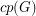denote the cardinality of a maximum cycle packing (collection of vertex disjoint cycles) and letdenote the cardinality of a minimum feedback vertex set (set of verticesso that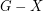is acyclic).

Conjecture   For every planar graph,.

Keywords: cycle packing; feedback vertex set; planar graph

## Chords of longest cycles ★★★

Author(s): Thomassen

Conjecture   Ifis a 3-connected graph, every longest cycle inhas a chord.

Keywords: chord; connectivity; cycle

## Hamiltonicity of Cayley graphs ★★★

Author(s): Rapaport-Strasser

Question   Is every Cayley graph Hamiltonian?

Keywords:

## Strong 5-cycle double cover conjecture ★★★

Author(s): Arthur; Hoffmann-Ostenhof

Conjecture   Let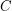be a circuit in a bridgeless cubic graph. Then there is a five cycle double cover ofsuch thatis a subgraph of one of these five cycles.

Keywords: cycle cover

## Decomposing an eulerian graph into cycles. ★★

Author(s): Hajós

Conjecture   Every simple eulerian graph onvertices can be decomposed into at mostcycles.

Keywords:

## Decomposing an eulerian graph into cycles with no two consecutives edges on a prescribed eulerian tour. ★★

Author(s): Sabidussi

Conjecture   Letbe an eulerian graph of minimum degree, and let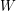be an eulerian tour of. Thenadmits a decomposition into cycles none of which contains two consecutive edges of.

Keywords:

## Every prism over a 3-connected planar graph is hamiltonian. ★★

Author(s): Kaiser; Král; Rosenfeld; Ryjácek; Voss

Conjecture   Ifis a-connected planar graph, then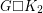has a Hamilton cycle.

Keywords:

## 4-connected graphs are not uniquely hamiltonian ★★

Author(s): Fleischner

Conjecture   Every-connected graph with a Hamilton cycle has a second Hamilton cycle.

Keywords:

## Hamilton decomposition of prisms over 3-connected cubic planar graphs ★★

Author(s): Alspach; Rosenfeld

Conjecture   Every prism over a-connected cubic planar graph can be decomposed into two Hamilton cycles.

Keywords: